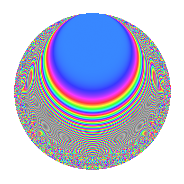# Properties

 Label 17.5.eLevel 17 Weight 5 Character orbit e Rep. character $$\chi_{17}(3,\cdot)$$ Character field $$\Q(\zeta_{16})$$ Dimension 40 Newform subspaces 1 Sturm bound 7 Trace bound 0

# Related objects

## Defining parameters

 Level: $$N$$ $$=$$ $$17$$ Weight: $$k$$ $$=$$ $$5$$ Character orbit: $$[\chi]$$ $$=$$ 17.e (of order $$16$$ and degree $$8$$) Character conductor: $$\operatorname{cond}(\chi)$$ $$=$$ $$17$$ Character field: $$\Q(\zeta_{16})$$ Newform subspaces: $$1$$ Sturm bound: $$7$$ Trace bound: $$0$$

## Dimensions

The following table gives the dimensions of various subspaces of $$M_{5}(17, [\chi])$$.

Total New Old
Modular forms 56 56 0
Cusp forms 40 40 0
Eisenstein series 16 16 0

## Trace form

 $$40q - 8q^{2} - 8q^{3} - 8q^{4} - 8q^{5} - 8q^{6} - 8q^{7} - 8q^{8} - 8q^{9} + O(q^{10})$$ $$40q - 8q^{2} - 8q^{3} - 8q^{4} - 8q^{5} - 8q^{6} - 8q^{7} - 8q^{8} - 8q^{9} + 376q^{10} + 112q^{11} - 776q^{12} - 416q^{13} - 776q^{14} - 704q^{15} + 256q^{17} + 2032q^{18} + 688q^{19} + 2680q^{20} + 2032q^{21} + 760q^{22} - 176q^{23} + 1672q^{24} - 2600q^{26} - 2600q^{27} - 7448q^{28} - 3368q^{29} - 9800q^{30} - 3720q^{31} - 2400q^{32} + 4280q^{34} + 4208q^{35} + 11960q^{36} + 7416q^{37} + 16720q^{38} + 15624q^{39} + 20280q^{40} + 2656q^{41} - 6392q^{42} - 7512q^{43} - 31592q^{44} - 23368q^{45} - 25752q^{46} - 10208q^{47} - 14080q^{48} - 3112q^{49} + 3224q^{51} + 12784q^{52} + 24424q^{53} + 51672q^{54} + 26648q^{55} + 40432q^{56} + 10352q^{57} - 4336q^{58} - 3176q^{59} - 38896q^{60} - 24600q^{61} - 39248q^{62} - 55664q^{63} - 45560q^{64} - 37928q^{65} - 29376q^{66} + 34912q^{68} + 46592q^{69} + 59536q^{70} + 21736q^{71} + 59824q^{72} + 28592q^{73} + 15976q^{74} + 46168q^{75} - 9280q^{76} + 2392q^{77} - 6048q^{78} - 15912q^{79} - 47640q^{80} - 48696q^{81} - 58368q^{82} - 27296q^{83} + 18872q^{85} + 74336q^{86} + 38536q^{87} + 55608q^{88} - 1232q^{89} - 24136q^{90} - 7800q^{91} - 27032q^{92} - 32232q^{93} - 37096q^{94} - 49640q^{95} - 79696q^{96} - 12392q^{97} - 76304q^{98} + 6056q^{99} + O(q^{100})$$

## Decomposition of $$S_{5}^{\mathrm{new}}(17, [\chi])$$ into newform subspaces

Label Dim. $$A$$ Field CM Traces $q$-expansion
$$a_2$$ $$a_3$$ $$a_5$$ $$a_7$$
17.5.e.a $$40$$ $$1.757$$ None $$-8$$ $$-8$$ $$-8$$ $$-8$$

## Hecke characteristic polynomials

There are no characteristic polynomials of Hecke operators in the database